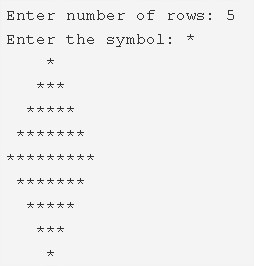# Diamond Star Pattern C Program – 4 Ways | C Patterns

C Program to print a diamond star pattern – In this article, we will detail in on the several means to print a diamond star pattern in C programming.

Suitable examples and sample programs have also been added so that you can understand the whole thing very clearly. The compiler has also been added with which you can execute it yourself.

The methods used in this particular piece are as follows:

• Using For Loop
• Using While Loop
• Using Do-While Loop

As you all know, a diamond is a very common figure used in the field of geometry. In order to socially and greedily accept a marriage, one of these is worn on the hands of their respective partners.

A diamond star pattern, as per the name of this article looks like this:As you can see, firstly, you need to enter the number of rows to print the respective shape.

The diamond shape will be printed accordingly. The number of rows is taken into consideration from both the sides of the centre of the diamond.

Thus, the multiple methods used in this blog to print a diamond star pattern in C programming are as follows:

## Using For Loop

1. Read the no.of rows and entered symbol, store the values into the variables n, ch.

2) The 1st for loop iterates through rows with the structure for(i=1;i<=n;i++).

a) The 2nd for loop iterates through columns with the structure for(j=1;j<=n-i;j++).

This loop prints space.

b) The 3rd for loop iterates through columns with the structure for(j=1;j<=i*2-1;j++).

This loop prints symbol.

c) Cursor comes to the next line for each iteration of i value.

After all iterations of i, the first half part of the diamond star pattern will be printed.

3) The 4th for loop iterates through rows with the structure for(i=n-1;i>0;i–).

a) The 5th for loop iterates through columns with the structure for(j=1;j<=n-i;j++).

This loop prints space.

b) The 6th for loop iterates through columns with the structure for(j=1;j<=2*i-1;j++).

This loop prints symbol.

c) Cursor comes to next line for each iteration of i.

After completion of the 3rd step, the remaining half part of the diamond star pattern will be printed.

Output:

### Using While Loop

1. i=1, The 1st while loop with the condition i<=n, iterates through rows until the condition becomes false.

a) j=1, The 2nd while loop with the condition j<=n-i, iterates through columns until the condition becomes false.

This loop prints space.j value increased by 1. The 2nd while loop checks the condition again.

b) j=1, The 3rd while loop with the condition j<=i*2-1, iterates through columns until the condition becomes false.

This loop prints symbol.j value increased by 1. The 3rd loop again checks the condition.

c) Cursor comes to next line, i value increased by 1. The 1st while loop again checks the condition.

After all these steps the first half part of the diamond star pattern will be printed.

2) i=n-1, The 4th while loop with the condition i>0, iterates through rows until the condition becomes false.

a) j=1, The 5th while loop with the condition j <= n-i ,iterates through columns until the condition becomes false.

This loop prints space. j value increased by 1. The 5th loop again checks the condition.

b) j=1, the 6th while loop with the condition j< = i*2-1, iterates through columns until the condition becomes false.

This loop prints symbol. j value increased by 1. The 6th loop again checks the condition.

c) Cursor comes to next line.i value decreased by 1. The 4th while loop again checks the condition.

After all these steps the remaining half part of the diamond star pattern will be printed.

Output:

#### Using Do-While Loop – Diamond Star Pattern C

1. i=1, the 1st do-while loop iterates through rows.

a) j=1, The 2nd do-while loop iterates through columns.

It prints space,j value increased by 1.

Checks the condition j<=n-i+1. If the loop iterates again. Repeats until the condition becomes false.

b) j=1, The 3rd do-while loop iterates through columns.

It prints symbol.j value increased by 1.

Checks the condition j<=i*2-1. If the condition is true then loop iterates again. Repeats until the condition becomes false.

c) Cursor comes to next line.i value increased by 1. Checks the condition i<=n. If the condition is true then loop iterates again.The 1st do-while loop iterates until the condition becomes false.

After all iterations of the above loops, The half part of the diamond star pattern will be printed.

2) i=n-1, The 4th do-while loop iterates through rows.

a) The 5th do-while loop iterates through columns.

This loop prints space.j value increased by 1.

Checks the condition j<=n-i+1. If the condition is true, loop iterates again. Repeats until the condition fails.

b) The 6th do-while loop iterates through columns.

This loop prints symbol.j value increased by 1.

Checks the condition j<=i*2-1. If the condition is true, loop iterates again. Repeats until the condition fails.

c) Cursor comes to next line.i value decreased by 1. Checks the condition i>0. If the condition is true, the 4th loop iterates again. Repeats until the condition becomes false.

Output:
x

## C Program To Check If Alphabet, Digit or Special Character | C Programs

C program to check for an alphabet, digit or special character – In this article, ...